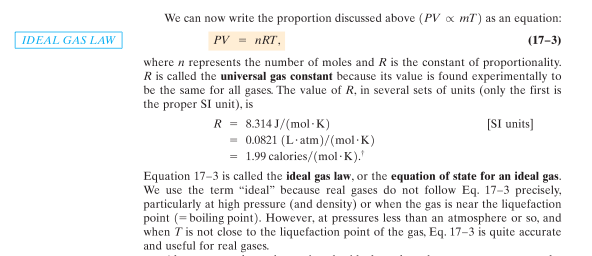# Problem: On the page 466 of the text the Ideal Gas Law is given as PV = nRT, why are there 3 different R's given?R = 8.314 J/mol•KR = 0.0821 (L•atm)/(mol•K)R = 1.99 calories/(mol•K)What the book says Page 466:

###### FREE Expert Solution

From the ideal gas law:

PV = nRT

The units of PV can either be Joule or L•atm

n is in mol.

T is in K.

R = PV/nT

R is in J/mol•K

The standard value of R is 8.314 J/mol•K.

This can be converted to the other two units, hence getting the three values of R.

1 atm = 101325 Pa
1 L = 10-3 m3
1 atm•L = 101.325 J
1J = 1/101.325 atm•L
1 J = 0.00987 atm•L

1 cal = 4.184 J

84% (215 ratings)###### Problem Details

On the page 466 of the text the Ideal Gas Law is given as PV = nRT, why are there 3 different R's given?

R = 8.314 J/mol•K

R = 0.0821 (L•atm)/(mol•K)

R = 1.99 calories/(mol•K)

What the book says Page 466: